# Physics - Electromagnetism - Mutual Inductance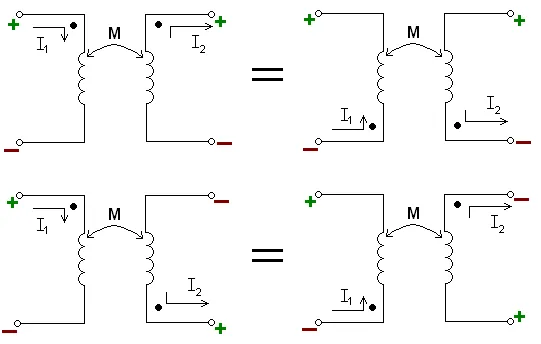Image source:## Introduction

Hello it's a me again Drifter Programming! Today we continue with Electromagnetism to get into Mutual Inductance which is a very interesting topic! So, without further do, let's get straight into it!

## Mutual Inductance

Until now we saw that an conductor generates an induced emf and current within itself as the result of electromagnetic induction, which is caused by a changing magnetic field around it. When this emf is induced from the same circuit to itself then this effect is called Self-Induction (L) something that we will cover next time...

The so called Mutual Inductance is about the interaction of a coil's magnetic field on another coil as it induces voltage in the adjacent coil. When the emf is induced into an adjacent coil within the same magnetic field, the emf is said to be induced magntetically, inductively or by Mutual Induction, symbolized as M. When two or more coils are magnetically linked together by a common magnetic flux they are said to have the property of Mutual Inductance. We can define mutual induction as the current flowing in one coil that induces a voltage in an adjacent coil. You can clearly see that I said the same thing with "different" words, so that you understand it better :P

Mutual Inductance has a lot of applications that we will cover in a bit, but is also a bad thing cause "stray" or "leakage" inductance from a coil can interfere with the operation of another adjacent component. To avoid this electromagnetic induction, some form of electrical screening to a ground potential may be required.

So, let's now get into the things that this mutual inductance depends on. The magnitude depends on the relative positioning of the two coils. By that I mean the physical distance that the coils are apart. When the distance is small more magnetic flux generated by the first coil will interact with the other coil, causing a large amount of mutual inductance. When the two coils are farther part and at different angels the amount of induced magnetic flux is much weaker then before and so the angle also plays a very important role. Another way of increasing the mutual inductance is by increasing the number of turns of either coil (transformer). A soft iron core or common soft iron core unity coupling can also minimize the losses due to the leakage of flux, something that shows us that the relative permeability also plays an important role.

We can summarize all this in the following equation: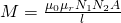Where:

• μ0 is the permeability of free space (4π x 10^-7)
• µr is the relative permeability of the soft iron core (or other material placed in-between)
• N is in the number of coil turns (for each coil separately)
• A is in the cross-sectional area in m^2
• l is the coils length in meters
Note that a perfect flux linkage was supposed between the two coils!

Magnetic inductance is a purely geometric quantity and depends only on the size, number of turns, relative position and relative orientation of the two circuits. In SI units mutual inductance is calculate in Henries (H) where: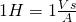The most typical unit used in experiments is the milli-henry (mH).

Note that the actual currents flowing through the wires don't affect the mutual inductance!

Let's now use M for any two arbitrary conducting circuits!

Consider two conducting circuits, labelled 1 and 2. A current I1 is flowing around circuit 1 and generating a magnetic field B1, which gives rise to a magnetic flux Φ2 linking it to circuit 2. Doubling the current would double the magnetic field and therefore flux on the second circuit. This conclusion shows us the linearity of the laws of magnetostatics. Having no current (I1 = 0) of course no magnetic flux is applied on circuit 2. The magnetic flux Φ2 is directly proportional to the current and mutual inductance as following:where M21 is the mutual inductance of circuit 2 with respect to circuit 1.

Similarly the flux Φ1 in circuit 1, because of the current I2 flowing around circuit 2 is:Mathematically we can prove that M = M12 = M21, something that I will avoid covering :P

Let's consider that the current flowing through circuit 1 changes by an amount dI1 in time interval dt. Therefore the magnetic flux changes by an amount of dΦ2 = MdI1 and according to Faraday's law the following amount of emf is being generated around the second circuit:Likewise the emf generated around the first circuit, when the current flowing through circuit 2 change by an amount of dI2 in time interval dt causing a magnetic flux change of dΦ1 = MdI, is:Note that there is no direct physical coupling between the two circuits. The coupling is due entirely to the magnetic field generated by the currents flowing around the circuits.

## Examples/Applications

Let's now get into some applications!

### Tesla coil

Consider a tesla coil, which is build up of a coil of N1 turns and a coil of N2 turns with a length of l and cross-sectional area A. Consider that a current i1 is flowing through the first coil (coil 1) and that a current i2 is flowing through coil 2. Let's get into the induced emf in coil 1 from coil 2...

The magnetic field due to coil 1 is: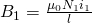The mutual inductance is: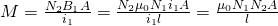The induced emf in coil 1 from coil 2 is: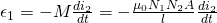### Transformer

A transformer is build up of two circuits called primary (actual energy supplier) and secondary and a iron core. When more current flows in the secondary of a transformer as it supplies more power, then more current must flow in the primary circuit as well, cause it's supplying the energy. This coupling between the primary and secondary is most conveniently described in terms of mutual inductance.

For ideal coupling the mutual inductance becomes:Applying the voltage law to both circuits of a transformer we get: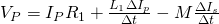The mutual inductance actas as the voltage source for the secondary circuit as:A transformer is the most common application of mutual inductance!

### REFERENCES:

Mathematical equations that I had in this post where drawn using quicklatex!

### Electric fields:

Getting into Electromagnetism -> electromagnetim, electric charge, conductors, insulators, quantization

Coulomb's law with examples -> Coulomb's law, superposition principle, Coulomb constant, how to solve problems, examples

Electric fields and field lines -> Electric fields, Solving problems around Electric fields and field lines

Electric dipoles -> Electric dipole, torque, potential and field

Electric charge and field Exercises -> examples in electric charges and fields

### Electric flux:

Electric flux and Gauss's law -> Electric flux, Gauss's law

Applications of Gauss's law (part 1) -> applying Gauss's law, Gauss applications

Applications of Gauss's law (part 2) -> more Gauss applications

Electric flux exercises -> examples in electric flux and Gauss's law

### Electric potential:

Electric potential energy -> explanation of work-energy, electric potential energy

Calculating electric potentials -> more stuff about potential energy, potential, calculating potentials

Millikan's Oil Drop Experiment -> Millikan's experiment, electronvolt

Cathode ray tubes explained using electric potential -> cathode ray tube explanation

Electric potential exercises (part 1) -> applications of potential

Electric potential exercises (part 2) -> applications of potential gradient, advanced examples

### Capacitance:

Capacitors (Condensers) and Capacitance -> Capacitors, capacitance, calculating capacitance

How to solve problems around Capacitors -> combination, solving problems, simple example

Electric field energy and density -> Electric field energy, energy density

Dielectric materials -> Dielectrics, dielectric constant, permittivity and strength, how to solve problems

Electric capacitance exercises -> examples in capacitance, energy density and dielectrics

### Current, resistance and EMF:

Electric current -> Electric current, current density

Electrical resistivity and conductivity -> Electrical resistivity, conductivity, thermal coefficient of resistivity, hyperconductivity

Electric resistance -> Resistance, temperature, resistors

Electromotive Force (EMF) and Internal resistance -> Electromotive force, internal resistance

Power and Wattage of Electronic Circuits -> Power in general, power/wattage of electronic circuits

Electric current, resistance and emf exercises -> exampes in all those topics

### Direct current (DC) circuits:

Resistor Combinations -> Resistor combinations, how to solve problems

Kirchhoff's laws with applications -> Kirchhoff's laws, how to solve problems, applications

Electrical measuring instruments -> what are they?, types list, getting into some of them, an application

Electronic circuits with resistors and capacitors (R-C) -> R-C Circuit, charging, time constant, discharging, how to apply

RC circuit exercises -> examples in Kirchhoff, charging, discharging capacitor with/without internal resistance

### Magnetic field and forces:

Magnetic fields -> Magnetism, Magnetic field

Magnetic field lines and Gauss's law of Magnetism -> magnetic field lines, mono- and dipoles, Flux, Gauss's law of magnetism

The motion of charged particles inside of a magnetic field -> straight-line, spiral and helical particle motion

Applications of charged particle motion -> CERN, Cyclotrons, Synchrotrons, Cavity Magetron, Mass Spectrometry and Magnetic lens

Magnetic force applied on Current-Carrying Conductors -> magnetic force on current-carrying conductors/wires, proofs

Magnetic force and torque applied on current loops (circuits) -> magnetic force on current loops, magnetic moment and torque

Explaining the Physics behind Electromotors -> tesla, history and explaining the physics behind them

Magnetic field exercises -> examples in magnetic force, magnetic flux, particle motion and forces/torque on current-carrying conductors

### Magnetic field sources:

Magnetic field of a moving charged particle -> moving charge, magnetic field, force between parallel charged particles

Magnetic field of current-carrying conductors -> magnetic field of current, Biot-Savart law

Force between parallel conductors and the magnetic field of a current loop-> force between parallel conductors, magnetic field of current loop

Ampere's law and Applications -> Ampere's law, applications

Magnetic materials -> Magnetic materials, classification and types, material examples

Displacement current -> Displacement current, Extension of Ampere's law

Exercises in Magnetic field sources -> examples all around magnetic field sources

### Electromagnetic Induction:

Motional Electromotive Force (Emf) -> Motional Emf, Faraday's law and motional emf, generalization

Lenz's law and Induced Electric fields -> Lenz's law, Induced Electric Fields

Eddy Currents and Applications -> Εddy currents, applications (brakes, testing, others)

Maxwell's equations -> What they are, each equation analyzed separately

Electromagnetic Induction exercises -> examples all around Electromagnetic Induction

And this is actually it for today's post! Next time in Physics we will get into Self Induction and maybe even Magnetic Energy...

Bye!

H2
H3
H4
3 columns
2 columns
1 column